GRE Subject Test: Chemistry : Boiling Point

Example Questions

Example Question #1 : Thermodynamics And Phases

Two moles of sodium chloride (NaCl) are added to 1kg of a mystery solvent. The addition of the NaCl caused an increase of 6K to the solvent's boiling point.

Based on this information, what is the boiling constant for the solvent?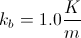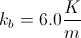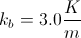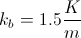Explanation:

In order to solve this problem, we can use the boiling point elevation equation: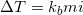.

We know the temperature change, we can compute molality from the given information, and we know the van't Hoff factor (expected to be 2 in this scenario due to NaCl becoming 2 ions in solution). We can calculate the boiling point constant for the solvent.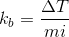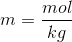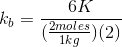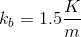Example Question #1 : Boiling Point

What is the boiling point of a solution composed of three liters of water and 50 grams of sodium chloride?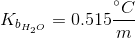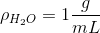The molar mass of sodium chloride is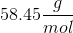.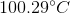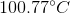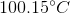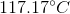Explanation:

We can use the boiling point elevation equation in order to determine the new boiling point once the salt has been added: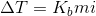Since sodium chloride will form two ions for each molecule in solution, the value for the van't Hoff factor will be 2. In addition, the mass of the water in the solution will be 3 kilograms, which can be determined by using the density of water.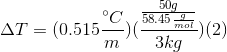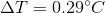Since water boils at 100 degrees Celsius, this means that the final boiling point of the solution is 100.29 degrees Celsius.

All GRE Subject Test: Chemistry Resources